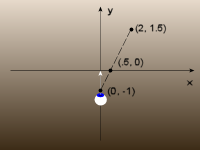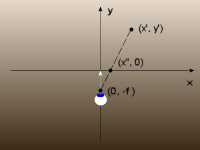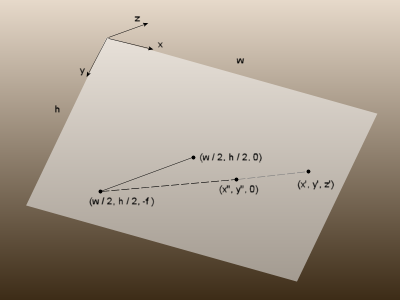# Bits & Bytes

## Perspective Projections in 3D with Actionscript 3.0

The most fundamental transformation in 3D graphics is the perspective transformation that projects points in 3D space onto the 2D image plane. In Actionscript, this is referred to as a Perspective Projection. This transformation is absolutely essential to creating an image from a 3D model. Most models are composed of sets of points. So, we will show how to project a single 3D point onto a 2D image. Once you know how to project a point, you can project virtually any complex object in 3D. For example, you can project a polygon by projecting each vertex, individually. Once the vertices are projected, you can connect them to create the projected polygon.Before we get into 3D, we want to consider how we can project a point in 2D onto a 1D line segment. This will demonstrate the essence of the problem and make the 3D case much easier to understand. So, we begin with 2D plane, and we want to project it onto the x-axis or the line y = 0. Every perspective projection has a viewpoint, which represents the observer’s eye. In this case, we set the viewpoint at (0, -1) and say that we are looking down the y-axis in the direction in which y is increasing (as shown by the white arrow). Now any point in the 2D can be projected onto our 1D image line given by the x-axis. For example, the point P’ = (1.5, 2) gets projected, by triangle similarity, onto the x-axis at P” = (.5, 0), where

x” = x’*(1/(2 + 1))

= 1.5*(1/3)

= .5

In general, a point (x’, y’) is transformed to (x”, 0) by x” = x’*(1/(y’ + 1)).This result can be further generalized by allowing the viewpoint to be anywhere on the negative y-axis. Here’s we have the viewpoint at (0, -f), and f is refered to as the focal length. Here, the point (x’, y’) is transformed to (x”, 0) by x” = x’*(f/(y’ + f)) in much the same way as before.Unlike the x-axis, images have finite length. Here, we used the interval [-w/2, w/2] to represent a one dimensional image with its width equal to w. If we project a point to the x-axis and it falls outside of this interval, it will not show up in our image. In this case, we say that the point is clipped. However, the finite image size presents us with an opportunity to define the field of view. The field of view is the angle θ defined at the viewpoint that encompasses the image width. By trigonometry, we have tan(θ/2) = (w/2)/f. So, the focal length, f, can be written in terms of the image width, w, and field of view like this f = w/(2*tan(θ/2)).

Next, we move to three dimensions and the default case for Actionscript. In this case, our origin is positioned in the upper-left corner of our image with the positive z-axis pointing into the screen. The image width and height are given by the stage object, and we will refer to them simply as w and h, respectively. With this, we have viewpoint located in the default position at (w/2, h/2, -f). The point (w/2, h/2) defines the vanishing point of the image and is called the projection center in Actionscript. By default, the field of view is 55 degrees, and the focal length is calculated from the field of view as f = w/(2*tan(θ/2)), which follows from the fact that tan(θ/2) = w/(2*f). In 3D, the projection of the 3D point P’ = (x’, y’, z’) to P” = (x”, y”, 0) in the 2D image space defined by the xy-plane or z = 0 is accomplished with the following equations

x” = w/2 + (x’ – w/2)*(z’/(z’ + f))

y” = h/2 + (y’ – h/2)*(z’/(z’ + f))In Actionscript, perspective transformations are accomplished by the PerspectiveProjection class, which has three members named: fieldOfView, focalLength, and projectionCenter. These properties correspond to the field of view, the focal length, and the projection center, respectively.

Keep in mind that the focal length and the field of view are not independent, but are related by the formula above. So, changing the value of one will change the other. The field of view is not used in the projection equations, but it is used as a convenient way to understand how projections work and set the focal length.

By: Michael Hall